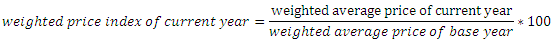# Weighted price index

The price index constructed by giving different weights to different commodities is called weighted price index. Weights are given on the basis of regularity in consumption and the amount spent and the number of consumers. The commodities consumed by all or majority of consumers spending large amount of income is given more weights and vive versa. The average price is calculated dividing the sum of weighted price by sum of weights.

weighted average price = sum of weighted prices / sum of weights
The major methods of weighted price index are fisher’s method, Laspeyre’s method, Pasche’s method etc. Weighted price index is calculated underWe can explain it with the help of table as following

 commodities Weight (W) Price in 2011 (P1) P1*W Price in 2012 (P2) P2*W Rice 10 Rs 35 Rs 350 Rs 40 Rs 400 Pulses 4 Rs 80 Rs 320 Rs 95 Rs 380 Clothes 3 Rs 250 Rs 750 Rs 275 Rs. 825 Vegetables 5 Rs 50 Rs  250 Rs 70 Rs 350 Firewood 2 Rs 100 Rs 200 Rs 150 Rs 300 total 24 Rs 515 Rs 1870 Rs 630 Rs 2255 Average 77.92 93.96 Price index 100 120.58

In the above table, we have taken only 5 commodities. Sum of the weighted prices of the base year 2011 is Rs 1870. The sum of weighted price of 2012 is Rs 2255. If we divide these sums of weighted prices by the sum of weights we obtain average price of the year2011 as Rs 77.92 and of the year 2012 Rs 93.95. Indexing price level of base year as 100 we can obtain the price index of the year 2012 as 1220.58. There is said to be rise in price level by 20.58%.